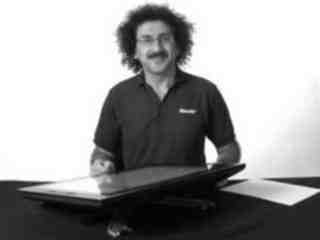Raffi Hovasapian

Potential Functions, Conclusion & Summary

Slide Duration:

Section 1: Vectors
Points & Vectors

28m 23s

Intro
0:00
Points and Vectors
1:02
A Point in a Plane
1:03
A Point in Space
3:14
Notation for a Space of a Given Space
6:34
Introduction to Vectors
9:51
14:51
Example 1
16:52
18:24
Example 2
21:01
Two More Properties of Vector Addition
24:16
Multiplication of a Vector by a Constant
25:27
Scalar Product & Norm

30m 25s

Intro
0:00
Scalar Product and Norm
1:05
Introduction to Scalar Product
1:06
Example 1
3:21
Properties of Scalar Product
6:14
Definition: Orthogonal
11:41
Example 2: Orthogonal
14:19
Definition: Norm of a Vector
15:30
Example 3
19:37
Distance Between Two Vectors
22:05
Example 4
27:19
More on Vectors & Norms

38m 18s

Intro
0:00
More on Vectors and Norms
0:38
Open Disc
0:39
Close Disc
3:14
Open Ball, Closed Ball, and the Sphere
5:22
Property and Definition of Unit Vector
7:16
Example 1
14:04
Three Special Unit Vectors
17:24
General Pythagorean Theorem
19:44
Projection
23:00
Example 2
28:35
Example 3
35:54
Inequalities & Parametric Lines

33m 19s

Intro
0:00
Inequalities and Parametric Lines
0:30
Starting Example
0:31
Theorem 1
5:10
Theorem 2
7:22
Definition 1: Parametric Equation of a Straight Line
10:16
Definition 2
17:38
Example 1
21:19
Example 2
25:20
Planes

29m 59s

Intro
0:00
Planes
0:18
Definition 1
0:19
Example 1
7:04
Example 2
12:45
General Definitions and Properties: 2 Vectors are Said to Be Paralleled If
14:50
Example 3
16:44
Example 4
20:17
More on Planes

34m 18s

Intro
0:00
More on Planes
0:25
Example 1
0:26
Distance From Some Point in Space to a Given Plane: Derivation
10:12
Final Formula for Distance
21:20
Example 2
23:09
Example 3: Part 1
26:56
Example 3: Part 2
31:46
Section 2: Differentiation of Vectors
Maps, Curves & Parameterizations

29m 48s

Intro
0:00
Maps, Curves and Parameterizations
1:10
Recall
1:11
Looking at y = x2 or f(x) = x2
2:23
Departure Space & Arrival Space
7:01
Looking at a 'Function' from ℝ to ℝ2
10:36
Example 1
14:50
Definition 1: Parameterized Curve
17:33
Example 2
21:56
Example 3
25:16
Differentiation of Vectors

39m 40s

Intro
0:00
Differentiation of Vectors
0:18
Example 1
0:19
Definition 1: Velocity of a Curve
1:45
Line Tangent to a Curve
6:10
Example 2
7:40
Definition 2: Speed of a Curve
12:18
Example 3
13:53
Definition 3: Acceleration Vector
16:37
Two Definitions for the Scalar Part of Acceleration
17:22
Rules for Differentiating Vectors: 1
19:52
Rules for Differentiating Vectors: 2
21:28
Rules for Differentiating Vectors: 3
22:03
Rules for Differentiating Vectors: 4
24:14
Example 4
26:57
Section 3: Functions of Several Variables
Functions of Several Variable

29m 31s

Intro
0:00
Length of a Curve in Space
0:25
Definition 1: Length of a Curve in Space
0:26
Extended Form
2:06
Example 1
3:40
Example 2
6:28
Functions of Several Variable
8:55
Functions of Several Variable
8:56
General Examples
11:11
Graph by Plotting
13:00
Example 1
16:31
Definition 1
18:33
Example 2
22:15
Equipotential Surfaces
25:27
Isothermal Surfaces
27:30
Partial Derivatives

23m 31s

Intro
0:00
Partial Derivatives
0:19
Example 1
0:20
Example 2
5:30
Example 3
7:48
Example 4
9:19
Definition 1
12:19
Example 5
14:24
Example 6
16:14
20:26
Higher and Mixed Partial Derivatives

30m 48s

Intro
0:00
Higher and Mixed Partial Derivatives
0:45
Definition 1: Open Set
0:46
Notation: Partial Derivatives
5:39
Example 1
12:00
Theorem 1
14:25
Now Consider a Function of Three Variables
16:50
Example 2
20:09
Caution
23:16
Example 3
25:42
Section 4: Chain Rule and The Gradient
The Chain Rule

28m 3s

Intro
0:00
The Chain Rule
0:45
Conceptual Example
0:46
Example 1
5:10
The Chain Rule
10:11
Example 2: Part 1
19:06
Example 2: Part 2 - Solving Directly
25:26
Tangent Plane

42m 25s

Intro
0:00
Tangent Plane
1:02
Tangent Plane Part 1
1:03
Tangent Plane Part 2
10:00
Tangent Plane Part 3
18:18
Tangent Plane Part 4
21:18
Definition 1: Tangent Plane to a Surface
27:46
Example 1: Find the Equation of the Plane Tangent to the Surface
31:18
Example 2: Find the Tangent Line to the Curve
36:54
Further Examples with Gradients & Tangents

47m 11s

Intro
0:00
Example 1: Parametric Equation for the Line Tangent to the Curve of Two Intersecting Surfaces
0:41
Part 1: Question
0:42
Part 2: When Two Surfaces in ℝ3 Intersect
4:31
Part 3: Diagrams
7:36
Part 4: Solution
12:10
Part 5: Diagram of Final Answer
23:52
Example 2: Gradients & Composite Functions
26:42
Part 1: Question
26:43
Part 2: Solution
29:21
Example 3: Cos of the Angle Between the Surfaces
39:20
Part 1: Question
39:21
Part 2: Definition of Angle Between Two Surfaces
41:04
Part 3: Solution
42:39
Directional Derivative

41m 22s

Intro
0:00
Directional Derivative
0:10
Rate of Change & Direction Overview
0:11
Rate of Change : Function of Two Variables
4:32
Directional Derivative
10:13
Example 1
18:26
Examining Gradient of f(p) ∙ A When A is a Unit Vector
25:30
Directional Derivative of f(p)
31:03
33:23
Example 2
34:53
A Unified View of Derivatives for Mappings

39m 41s

Intro
0:00
A Unified View of Derivatives for Mappings
1:29
Derivatives for Mappings
1:30
Example 1
5:46
Example 2
8:25
Example 3
12:08
Example 4
14:35
Derivative for Mappings of Composite Function
17:47
Example 5
22:15
Example 6
28:42
Section 5: Maxima and Minima
Maxima & Minima

36m 41s

Intro
0:00
Maxima and Minima
0:35
Definition 1: Critical Point
0:36
Example 1: Find the Critical Values
2:48
Definition 2: Local Max & Local Min
10:03
Theorem 1
14:10
Example 2: Local Max, Min, and Extreme
18:28
Definition 3: Boundary Point
27:00
Definition 4: Closed Set
29:50
Definition 5: Bounded Set
31:32
Theorem 2
33:34
Further Examples with Extrema

32m 48s

Intro
0:00
Further Example with Extrema
1:02
Example 1: Max and Min Values of f on the Square
1:03
Example 2: Find the Extreme for f(x,y) = x² + 2y² - x
10:44
Example 3: Max and Min Value of f(x,y) = (x²+ y²)⁻¹ in the Region (x -2)²+ y² ≤ 1
17:20
Lagrange Multipliers

32m 32s

Intro
0:00
Lagrange Multipliers
1:13
Theorem 1
1:14
Method
6:35
Example 1: Find the Largest and Smallest Values that f Achieves Subject to g
9:14
Example 2: Find the Max & Min Values of f(x,y)= 3x + 4y on the Circle x² + y² = 1
22:18
More Lagrange Multiplier Examples

27m 42s

Intro
0:00
Example 1: Find the Point on the Surface z² -xy = 1 Closet to the Origin
0:54
Part 1
0:55
Part 2
7:37
Part 3
10:44
Example 2: Find the Max & Min of f(x,y) = x² + 2y - x on the Closed Disc of Radius 1 Centered at the Origin
16:05
Part 1
16:06
Part 2
19:33
Part 3
23:17
Lagrange Multipliers, Continued

31m 47s

Intro
0:00
Lagrange Multipliers
0:42
First Example of Lesson 20
0:44
Let's Look at This Geometrically
3:12
Example 1: Lagrange Multiplier Problem with 2 Constraints
8:42
Part 1: Question
8:43
Part 2: What We Have to Solve
15:13
Part 3: Case 1
20:49
Part 4: Case 2
22:59
Part 5: Final Solution
25:45
Section 6: Line Integrals and Potential Functions
Line Integrals

36m 8s

Intro
0:00
Line Integrals
0:18
Introduction to Line Integrals
0:19
Definition 1: Vector Field
3:57
Example 1
5:46
Example 2: Gradient Operator & Vector Field
8:06
Example 3
12:19
Vector Field, Curve in Space & Line Integrals
14:07
Definition 2: F(C(t)) ∙ C'(t) is a Function of t
17:45
Example 4
18:10
Definition 3: Line Integrals
20:21
Example 5
25:00
Example 6
30:33
More on Line Integrals

28m 4s

Intro
0:00
More on Line Integrals
0:10
Line Integrals Notation
0:11
Curve Given in Non-parameterized Way: In General
4:34
Curve Given in Non-parameterized Way: For the Circle of Radius r
6:07
Curve Given in Non-parameterized Way: For a Straight Line Segment Between P & Q
6:32
The Integral is Independent of the Parameterization Chosen
7:17
Example 1: Find the Integral on the Ellipse Centered at the Origin
9:18
Example 2: Find the Integral of the Vector Field
16:26
Discussion of Result and Vector Field for Example 2
23:52
Graphical Example
26:03
Line Integrals, Part 3

29m 30s

Intro
0:00
Line Integrals
0:12
Piecewise Continuous Path
0:13
Closed Path
1:47
Example 1: Find the Integral
3:50
The Reverse Path
14:14
Theorem 1
16:18
Parameterization for the Reverse Path
17:24
Example 2
18:50
Line Integrals of Functions on ℝn
21:36
Example 3
24:20
Potential Functions

40m 19s

Intro
0:00
Potential Functions
0:08
Definition 1: Potential Functions
0:09
Definition 2: An Open Set S is Called Connected if…
5:52
Theorem 1
8:19
Existence of a Potential Function
11:04
Theorem 2
18:06
Example 1
22:18
Contrapositive and Positive Form of the Theorem
28:02
The Converse is Not Generally True
30:59
Our Theorem
32:55
Compare the n-th Term Test for Divergence of an Infinite Series
36:00
So for Our Theorem
38:16
Potential Functions, Continued

31m 45s

Intro
0:00
Potential Functions
0:52
Theorem 1
0:53
Example 1
4:00
Theorem in 3-Space
14:07
Example 2
17:53
Example 3
24:07
Potential Functions, Conclusion & Summary

28m 22s

Intro
0:00
Potential Functions
0:16
Theorem 1
0:17
In Other Words
3:25
Corollary
5:22
Example 1
7:45
Theorem 2
11:34
Summary on Potential Functions 1
15:32
Summary on Potential Functions 2
17:26
Summary on Potential Functions 3
18:43
Case 1
19:24
Case 2
20:48
Case 3
21:35
Example 2
23:59
Section 7: Double Integrals
Double Integrals

29m 46s

Intro
0:00
Double Integrals
0:52
Introduction to Double Integrals
0:53
Function with Two Variables
3:39
Example 1: Find the Integral of xy³ over the Region x ϵ[1,2] & y ϵ[4,6]
9:42
Example 2: f(x,y) = x²y & R be the Region Such That x ϵ[2,3] & x² ≤ y ≤ x³
15:07
Example 3: f(x,y) = 4xy over the Region Bounded by y= 0, y= x, and y= -x+3
19:20
Polar Coordinates

36m 17s

Intro
0:00
Polar Coordinates
0:50
Polar Coordinates
0:51
Example 1: Let (x,y) = (6,√6), Convert to Polar Coordinates
3:24
Example 2: Express the Circle (x-2)² + y² = 4 in Polar Form.
5:46
Graphing Function in Polar Form.
10:02
Converting a Region in the xy-plane to Polar Coordinates
14:14
Example 3: Find the Integral over the Region Bounded by the Semicircle
20:06
Example 4: Find the Integral over the Region
27:57
Example 5: Find the Integral of f(x,y) = x² over the Region Contained by r= 1 - cosθ
32:55
Green's Theorem

38m 1s

Intro
0:00
Green's Theorem
0:38
Introduction to Green's Theorem and Notations
0:39
Green's Theorem
3:17
Example 1: Find the Integral of the Vector Field around the Ellipse
8:30
Verifying Green's Theorem with Example 1
15:35
A More General Version of Green's Theorem
20:03
Example 2
22:59
Example 3
26:30
Example 4
32:05
Divergence & Curl of a Vector Field

37m 16s

Intro
0:00
Divergence & Curl of a Vector Field
0:18
Definitions: Divergence(F) & Curl(F)
0:19
Example 1: Evaluate Divergence(F) and Curl(F)
3:43
Properties of Divergence
9:24
Properties of Curl
12:24
Two Versions of Green's Theorem: Circulation - Curl
17:46
Two Versions of Green's Theorem: Flux Divergence
19:09
Circulation-Curl Part 1
20:08
Circulation-Curl Part 2
28:29
Example 2
32:06
Divergence & Curl, Continued

33m 7s

Intro
0:00
Divergence & Curl, Continued
0:24
Divergence Part 1
0:25
Divergence Part 2: Right Normal Vector and Left Normal Vector
5:28
Divergence Part 3
9:09
Divergence Part 4
13:51
Divergence Part 5
19:19
Example 1
23:40
Final Comments on Divergence & Curl

16m 49s

Intro
0:00
Final Comments on Divergence and Curl
0:37
Several Symbolic Representations for Green's Theorem
0:38
Circulation-Curl
9:44
Flux Divergence
11:02
Closing Comments on Divergence and Curl
15:04
Section 8: Triple Integrals
Triple Integrals

27m 24s

Intro
0:00
Triple Integrals
0:21
Example 1
2:01
Example 2
9:42
Example 3
15:25
Example 4
20:54
Cylindrical & Spherical Coordinates

35m 33s

Intro
0:00
Cylindrical and Spherical Coordinates
0:42
Cylindrical Coordinates
0:43
When Integrating Over a Region in 3-space, Upon Transformation the Triple Integral Becomes..
4:29
Example 1
6:27
The Cartesian Integral
15:00
Introduction to Spherical Coordinates
19:44
Reason It's Called Spherical Coordinates
22:49
Spherical Transformation
26:12
Example 2
29:23
Section 9: Surface Integrals and Stokes' Theorem
Parameterizing Surfaces & Cross Product

41m 29s

Intro
0:00
Parameterizing Surfaces
0:40
Describing a Line or a Curve Parametrically
0:41
Describing a Line or a Curve Parametrically: Example
1:52
Describing a Surface Parametrically
2:58
Describing a Surface Parametrically: Example
5:30
Recall: Parameterizations are not Unique
7:18
Example 1: Sphere of Radius R
8:22
Example 2: Another P for the Sphere of Radius R
10:52
This is True in General
13:35
Example 3: Paraboloid
15:05
Example 4: A Surface of Revolution around z-axis
18:10
Cross Product
23:15
Defining Cross Product
23:16
Example 5: Part 1
28:04
Example 5: Part 2 - Right Hand Rule
32:31
Example 6
37:20
Tangent Plane & Normal Vector to a Surface

37m 6s

Intro
0:00
Tangent Plane and Normal Vector to a Surface
0:35
Tangent Plane and Normal Vector to a Surface Part 1
0:36
Tangent Plane and Normal Vector to a Surface Part 2
5:22
Tangent Plane and Normal Vector to a Surface Part 3
13:42
Example 1: Question & Solution
17:59
Example 1: Illustrative Explanation of the Solution
28:37
Example 2: Question & Solution
30:55
Example 2: Illustrative Explanation of the Solution
35:10
Surface Area

32m 48s

Intro
0:00
Surface Area
0:27
Introduction to Surface Area
0:28
Given a Surface in 3-space and a Parameterization P
3:31
Defining Surface Area
7:46
Curve Length
10:52
Example 1: Find the Are of a Sphere of Radius R
15:03
Example 2: Find the Area of the Paraboloid z= x² + y² for 0 ≤ z ≤ 5
19:10
Example 2: Writing the Answer in Polar Coordinates
28:07
Surface Integrals

46m 52s

Intro
0:00
Surface Integrals
0:25
Introduction to Surface Integrals
0:26
General Integral for Surface Are of Any Parameterization
3:03
Integral of a Function Over a Surface
4:47
Example 1
9:53
Integral of a Vector Field Over a Surface
17:20
Example 2
22:15
Side Note: Be Very Careful
28:58
Example 3
30:42
Summary
43:57
Divergence & Curl in 3-Space

23m 40s

Intro
0:00
Divergence and Curl in 3-Space
0:26
Introduction to Divergence and Curl in 3-Space
0:27
Define: Divergence of F
2:50
Define: Curl of F
4:12
The Del Operator
6:25
Symbolically: Div(F)
9:03
Symbolically: Curl(F)
10:50
Example 1
14:07
Example 2
18:01
Divergence Theorem in 3-Space

34m 12s

Intro
0:00
Divergence Theorem in 3-Space
0:36
Green's Flux-Divergence
0:37
Divergence Theorem in 3-Space
3:34
Note: Closed Surface
6:43
Figure: Paraboloid
8:44
Example 1
12:13
Example 2
18:50
Recap for Surfaces: Introduction
27:50
Recap for Surfaces: Surface Area
29:16
Recap for Surfaces: Surface Integral of a Function
29:50
Recap for Surfaces: Surface Integral of a Vector Field
30:39
Recap for Surfaces: Divergence Theorem
32:32
Stokes' Theorem, Part 1

22m 1s

Intro
0:00
Stokes' Theorem
0:25
Recall Circulation-Curl Version of Green's Theorem
0:26
Constructing a Surface in 3-Space
2:26
Stokes' Theorem
5:34
Note on Curve and Vector Field in 3-Space
9:50
Example 1: Find the Circulation of F around the Curve
12:40
Part 1: Question
12:48
Part 2: Drawing the Figure
13:56
Part 3: Solution
16:08
Stokes' Theorem, Part 2

20m 32s

Intro
0:00
Example 1: Calculate the Boundary of the Surface and the Circulation of F around this Boundary
0:30
Part 1: Question
0:31
Part 2: Drawing the Figure
2:02
Part 3: Solution
5:24
Example 2: Calculate the Boundary of the Surface and the Circulation of F around this Boundary
13:11
Part 1: Question
13:12
Part 2: Solution
13:56
Bookmark & Share Embed

## Copy & Paste this embed code into your website’s HTML

Please ensure that your website editor is in text mode when you paste the code.
(In Wordpress, the mode button is on the top right corner.)
×
• - Allow users to view the embedded video in full-size.
Since this lesson is not free, only the preview will appear on your website.

• ## Related Books

### Start Learning Now

Our free lessons will get you started (Adobe Flash® required).

### Membership Overview

• *Ask questions and get answers from the community and our teachers!
• Practice questions with step-by-step solutions.
• Track your course viewing progress.
• Learn at your own pace... anytime, anywhere!

### Potential Functions, Conclusion & Summary

Let F(x,y) = (y,x) be on an open plane S with a potential function f(x,y) = xy.
Let C be a path on F connecting (0,1) to (3, − 2). Find ∫C F .
• By the fundamental theorem of line integrals, ∫C F = PF(Q) − PF(P) where PF is the potential function for the vector field F with P and Q being points on C.
Let P = (0,1) and Q = (3, − 2), then ∫C F = f(3, − 2) − f(0,1) = − 6 − 0 = − 6.
Let F be a vector field and f(x,y) = 3xy − 2y + x be a potential function for F.
i) Determine ∫C F for the path on the open set S.
•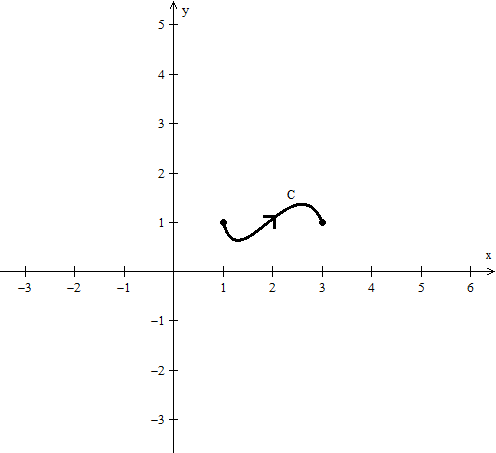• By the fundamental theorem of line integrals, ∫C F = PF(Q) − PF(P) where PF is the potential function for the vector field F with P and Q being points on C.
We then have P = (1,1) and Q = (3,1), and so ∫C F = f(3,1) − f(1,1) = 10 − 2 = 8.
Let F be a vector field and f(x,y) = 3xy − 2y + x be a potential function for F.
ii) Determine ∫C F for the path on the open set S.
• Note that C is just the change of orientation of our path.
•Since ∫C F = − ∫C F it follows that ∫C F = − 8.
Let F be a vector field and f(x,y) = 3xy − 2y + x be a potential function for F.
iii) Determine ∫C′ F for the path on the open set S. C′
•• Since a potential function f exists, ∫F is path independent. Note the orientation of our path C′.
Hence ∫C′ F = ∫C F = 8.
Let F be a vector field and f(x,y) = 3xy − 2y + x be a potential function for F.
iv) Determine ∫C0 F for the path C0 = C1 + C2 on the open set S.
•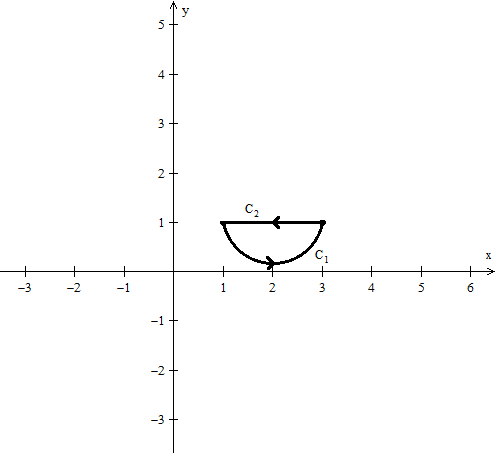• Since ∫F is path independent any closed path has ∫C F = 0.
Note that C0 = C1 + C2 form a closed path, that is the endpoint and starpoint are the same. Hence ∫C0 F = 0.
Let F be a vector field on an open plane S and C = C1 + C2 be described by: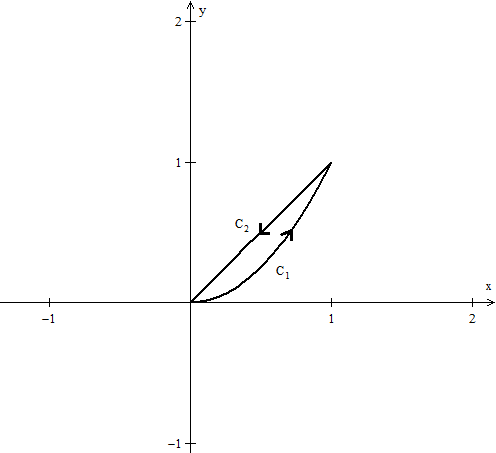If ∫C F = − 1, is there a potential function f for F?
Since ∫C F 0 along the closed path C, no potential function f exists for F.
Let F be a vector field on an open plane S and C = C1 + C2 + C3 + C4 be described by: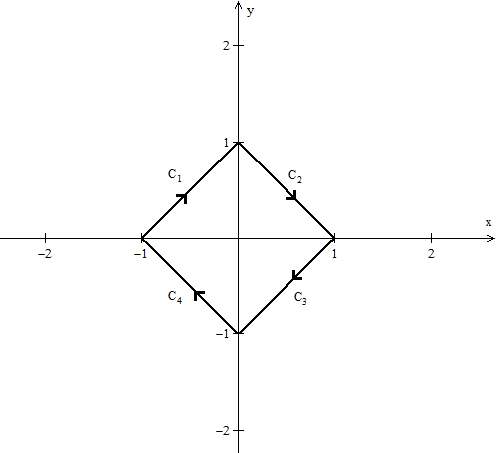and ∫C F = 0. For C′ be described by: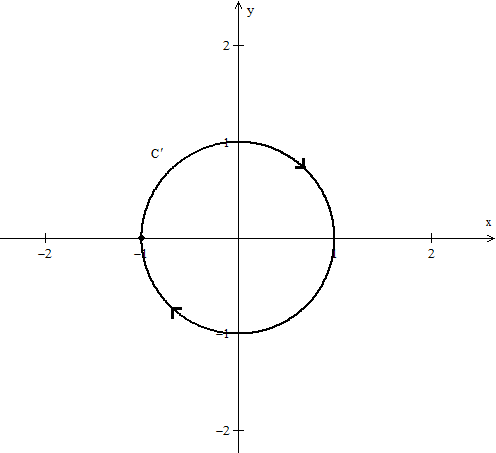Does ∫C′ F = 0?
• Note necessarily, just because one closed path yielded zero, that does not guarantee that every closed path is zero.
This is because we have not shown path independence, that is, we have not shown the existance of a potential function f for F.
Let F be a vector field on an open plane S and ∫C1 F = ∫C2 F = ∫C3 F + ∫C4 F be described by: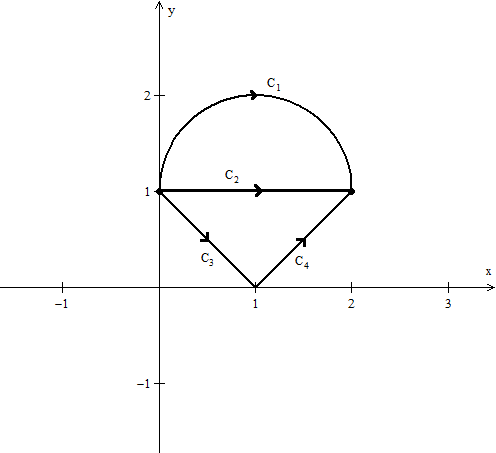Is there a potential function f for F?
• Yes, the fact that three different path integrals yield the same result make ∫F path independent.
So we are verified the existance of a potential function f for F.
Let F be a vector field. Explain why the following statement is false.
If ∫C F = 0 over an open and connected set, then F has a potential function f.
F has a potential function f if and only if every closed path yields ∫C F = 0.
Let F be a vector field. Explain why the following statement is false.
If ∫C F = k with k > 0 over an open and connected set, then F does not have a potential function f.
The statement is only true if C is a closed path.
Let F be a vector field. Explain why the following statement is false.
If F is an open and connected set, then ∫C F = 0 for every closed path C.
The statement is only true if F is a conservative vector field, that is there exist a potential function f for F.
Let F be a vector field. Explain why the following statement is false.
If F is on an open set, then ∫C F = ∫C1 F + ∫C2 F + ∫C3 F for C = C1 + C2 + C3
.
The set F lies on must not only be connected but C must be on the set as well.
Let F be a vector field. Explain why the following statement is false.
If F is on an open and connected set with ∫C F = 0, then ∫C F = 0.
The statement is only true if C is a closed path.

*These practice questions are only helpful when you work on them offline on a piece of paper and then use the solution steps function to check your answer.

### Potential Functions, Conclusion & Summary

Lecture Slides are screen-captured images of important points in the lecture. Students can download and print out these lecture slide images to do practice problems as well as take notes while watching the lecture.

• Intro 0:00
• Potential Functions 0:16
• Theorem 1
• In Other Words
• Corollary
• Example 1
• Theorem 2
• Summary on Potential Functions 1
• Summary on Potential Functions 2
• Summary on Potential Functions 3
• Case 1
• Case 2
• Case 3
• Example 2

### Transcription: Potential Functions, Conclusion & Summary

Hello and welcome back to educator.com and multivariable calculus.0000

Today we are going to close out, or round out the story on potential functions, and summarize everything so we have a nice set of things to follow in order to decide how potential functions behave.0004

Okay. So, let us get started. So, let us start off with a theorem, here.0017

So, we will let f be a vector field, let f be a vector field on an open set s -- excuse me -- an open set s and let pf be a potential function for f on s.0026

Okay... continue over here... let c be a path in s connecting the points p and q.0065

Then, the integral of f/c from point p to point q is equal to the potential function evaluated at q, the end point, minus the potential function evaluated at p, the beginning point.0087

Also, let us write this here -- also, this is the really interesting part in my opinion -- the integral is independent of the path.0109

Okay. I should actually have the path connecting p and q, I mean we know that it connects p and q, but let us just write it out anyways -- connecting p and q.0131

So, basically what this theorem says is that if you have this open set s and if you know that your particular vector field has a potential function, well instead of actually evaluating the integral itself, the way we did previously, evaluating the line integral, because in some cases it might be hard, you can just take the potential function, and evaluate the potential function at q and subtract the potential function at f.0142

This is pretty much the same thing as the fundamental theorem of calculus in single variable, this is just a version of it.0169

For some function, some vector field that emits a potential function, this is how we can actually calculate the integral instead of going into the line integral process.0175

What is also interesting is that the integral is independent of the path, so it does not matter if I get there via straight line or a curve, or if I bounce around all over the set and eventually get there.0184

It does not matter. The integral actually ends up being the same. That is extraordinary, absolutely extraordinary and a profound importance in physics.0196

Let me actually write out what this is so that you have it here.0206

In other words, the integral of a vector field which admits a potential function over a domain is equal to the difference of the potential function evaluated at the end points, and is path independent.0210

So, if this is p and this is q, if you are moving in that direction, integrating along that path, you are going to get the same value whether you go like this or whether you go like that, whether you go like that, it does not matter. The path does not matter.0282

The integral stays the same, simply because on this open set, the vector field admits a potential function.0309

So, the existence of a potential function is a very, very special kind of property that is related to the vector field under discussion.0315

Okay. So, let us go ahead and write out a corollary to this.0323

All of the previous hypotheses, let f be a vector field on an open set s which admits a potential function... okay, I will just write if previous hypotheses, then the integral of f around every closed path, every closed curve -- I will write path instead of curve -- in s = 0.0337

Because a closed path, you remember, the beginning point and the end point are the same. When you evaluate them of course you are going to get a - a, b - b, it is going to be 0.0381

Okay. So... and if there exists a closed path in s such that the integral of f does not equal 0, then f does not have a potential function.0393

This is the contra-positive version of the corollary. What we wrote... this part, this is the contra-positive.0424

So, if a vector field admits a potential function on an open set s, then the integral over every closed path in s, every closed path is going to be 0.0430

If you can find a closed path in s where the integral does not equal 0, that means the vector field does not have a potential function.0440

Again, it just depends on the direction we are working in. That is all this is -- okay... does not have a potential function -- you know what, let me not actually does not have a potential function, let me just write does not have a pf.0448

Okay. Let us do an example here. So, example 1.0468

So, in a previous lesson we had a vector field f which was equal to 4xy and 2x2.0473

Okay? That admitted a potential function, which ended up being 2x2y.0493

Well, let p be the point (-4,7) and let q be the point (3,-2) and c be any path connecting them.0501

Then, the integral of f over c is equal to, well, it is equal to the potential function evaluated at... let me see, what are our 2 points... q, right? it is the ending... so we are going from p to q.0533

So it is going to be the potential function evaluated at 3 - 2, minus the potential function evaluated at (-4,7).0550

There we go. Then when we actually do this evaluation, we end up with something like again, if I did my arithmetic correct... and by all means, please check my arithmetic but arithmetic is not important, it is the mathematics that is important.0565

I think you get something like -36 - 224, and I hope my number are right... -360... so this is the final answer, this is what is important, right here... this.0579

You do not actually have to go ahead and parameterize the path and solve the integral, you do not have to take the f(c(t)) · c'(t), evaluate that integral, just take... because it admits a potential function, you can just evaluate it as a potential function at the two end points and that is going to be your integral value.0596

Okay. So, let us see what we have. Now, as it turns out -- I will write it over here -- as it turns out, in this case, the converse of this theorem is also true.0615

So, remember that we said the converse if... if we have a theorem that says if a, then b, the converse is if b, then a, you just switch the places of b and a.0646

Different than the contra-positive, the contra-positive, if the positive is a, then b, the contra-positive is if not a, then not b.0656

Those two are equivalent, so, if I have a theorem, the contra-positive is automatically true.0663

In this particular case, the converse is also true, but it is not true just automatically. It actually has to be proven to be true.0670

Again, we are not going to through the proof, we are not interested in the details of the proof, we are interested in the results, but as long as you differentiate between the two.0677

A positive statement and its contrapositive come together. They are both, they are equivalent.0685

The converse may or may not be true, you have to check to see if it is. In this case it is, so let us go ahead and write it out. Let us go to the next page here.0690

It is always nice when converses are true, it always makes life a lot easier. It gives you two directions to work in depending on what your particular situation is.0702

Let f be a vector field on an open connected set -- you know, I am just going to go ahead and start presuming that the hypotheses are given -- okay, if the integral of f is path independent, then f admits a potential function.0713

In other words, a potential function exists. In other words, if you happen to have a vector field, and you happen to have two paths that you can check on two points connecting... two paths connecting two different points, and let us say they are reasonably easily parameterized, if you took the integrals and the integrals ended up being the same, you could conclude that there is a potential function.0744

You do not need anything else at that point. Again, it just depends what you have at your disposal.0767

All of these theorems are there as tools in your toolbox. You might be presented with a vector field in a particular kind of open set.0773

You can draw a conclusion. You might be presented with a vector field and a path that is easily parameterizable.0781

You might be presented with an integral value -- all kinds of things you can be presented with, we just want to present theorems that allow you to test when something has a potential function, when something does not, when you have to evaluate the line integral directly, when you have to use the potential function to evaluate it at different points, that is all that is going on here. That is why we are listing these theorems.0788

Okay, so, if f is path independent, then f admits a potential function. That is the converse of the other one. Now, let us see.0812

If the particular paths in this theorem are closed, we have the following version of the theorem.0824

Again, this is just the same theorem as this except for closed paths. We have the following version.0845

If, the integral of f = 0 for every closed path, then f has a potential function.0857

Now, the only reason that we gave this particular version of the theorem is for the sake of completeness. There is no way that you are going to test every single path.0889

There is an infinite number of paths, an infinite number of closed paths in an open set.0897

So, from a practical standpoint, this theorem has absolutely no value at all. From a theoretical standpoint, it does, of course.0901

But, again, for practical purposes, you cannot really use this in any reasonable way, because you cannot test every path.0909

Fortunately, we have other tools at our disposal to check to see whether a potential function exists.0915

Now, let us go ahead and summarize what we have got up to here. So, let me draw a line and let me go back to black.0921

So, summary on potential functions... summary on potential functions.0932

Okay. So, let us start off with let f = fg, I am only going to state it for 2-space, but of course it is valid for n-space, be a vector field on an open connected set.0946

Okay. On an open connected s -- let us actually give a name to this set.0977

Okay. Number 1. Our first test. If d2(f) = d1(g) -- I am sorry, if it does not equal... let me write this again here -- if d2(f) does not equal d1(g), then there does not exist a potential function.0984

Now, this is the same as df/dy not equaling dg/dx.1021

Capital D notation up here, regular standard notation, more common notation for partial derivatives down here.1033

So, if d2(f) does not equal d1(g), then there does not exist a potential function, we are done.1042

Number 2. Second possibility. If d2(f) does equal d1(g) and s is a rectangle or the entire plane, then there exists a potential function.1049

I will start abbreviating it that way, and we can find it and we can find the potential function by integrating one variable at a time.1086

Remember we did several examples of that by integrating one variable at a time and again, the only thing you have to do when you are actually doing that is just be careful because there are a lot of x's and y'x and z's floating around.1102

Lots of functions -- functions, vector fields -- you just have to keep track of everything. One variable at a time.1113

So, let us have... there is a third possibility... if d2(f) = d1(g) -- I always keep putting an i there, d1(g) -- but s is not a rectangle or the entire plane, then a potential function may or may not exist.1124

Okay. Let us do case 1. So, we move onto another test, another series of, you know, that is the thing. We have this toolbox, if one thing does not work, we move onto something else, and if something does not give us a conclusive answer, we move onto a finer -- you know -- a finer version. Something else that we can test.1166

That is what we do. We just run down the list of possibilities. So, if d2(f) = d1(g) but s is not a rectangle, then a potential function may or may not exist.1191

So, the first case is if there exists some closed path, some closed curve c such that the integral of f along c does not equal 0, then there does not exist a potential function.1202

Okay. So, this particular case says I take d2(f), it happens to equal d1(g), it is not a rectangle so I cannot conclude that there is a potential function, but if I can conclude that there is some potential path, some closed path where the integral does not equal 0, then I conclude that there is not a potential function on that domain.1229

Okay. Case 2. Now, if the integral of f around every closed path in s equals 0, then there exists a potential function.1251

I will put in parentheses... (not practical)... this one, again, strictly for theoretical purposes, you are going to have to find something else to test some other way of finding out whether a potential function exists.1281

Okay. Let us do case 3 here. Case 3. Okay. If s is, and this is the interesting one, is the entire plane from which the origin has been deleted... so if s is the entire plane from which the origin has been deleted, so our particular set s we have just taken out the origin -- deleted -- and c is the unit circle, and if the integral of f/c = 0, then their exists a potential function.1297

So, in this particular case, I happen to be dealing with a vector field that is not defined at the origin, so I actually have to remove the origin from consideration.1374

Now it is just the entire plane with the origin missing, and if I go ahead and integrate that vector field around the unit circle... just a basic unit circle, if I get an integral that is actually equal to 0, then I can conclude that a potential function exists.1385

This is actually pretty extraordinary. You are going to run across all kinds of vector fields, whether it be mathematics, engineering, or particularly in physics.1402

Simply by taking the origin out, that one point out, all of a sudden everything changes. That is really truly an extraordinary thing. that the existence of the origin as a point that is viable in our particular connected open set, it really just changes the nature of the function itself.1413

By removing the origin, everything changes. Again, absolutely fascinating, absolutely extraordinary.1434

Let us go ahead and do an example to round this out.1442

Example 2. Okay. So, we are going to let f(x,y)... we have seen this vector field before... equal -y/x2 + y2, and x/x2 + y2.1447

Now, c(t), so this is our vector field, our curve is going to be the unit circle, and sin(t)... and we are going to take t from 0 to 2pi, that is the unit circle, and s, our open set, is the entire plane because this vector is defined over the entire plane excluding the origin.1470

Because the point (0,0), this vector field is not defined, excluding the origin.1505

Well, let us go ahead and form f(c(t)), we are going to evaluate this directly. So, f(c(t)) = -sin(t)/1, right? because if I put cos(t) for x and sin(t) for y, cos2 + sin2 is 1, standard trigonometric identity, and this is going to be cos(t)/1.1513

Now c'(t) that equals -sin(t) and cos(t). Okay.1541

So, when we form f(c(t)) · c'(t), when I dot these two I end up getting sin2(t), right? -sin(t) - sin(t), sin2(t) + cos2(t) = 1.1551

Let us see here. Is equal to 1... so now we are going to... let me do this on the next page... so now the integral of f, the integral of our vector field over our unit circle is equal to the integral from 0 to 2pi of just 1 × dt, which is dt.1574

That is going to equal t from 0 to 2pi, which equals 2pi.1597

2pi does not equal 0. This implies that there does not exist a potential function.1604

Let me write "there does not exist a potential function" for this particular vector field on this particular open set, the plane with the origin deleted.1618

If it were equal to 0, then we could conclude that a potential function exists. In this particular case, potential function does not exist for this vector field.1632

Now, if our open set changes, a potential function for this vector field may actually exist.1641

Again, do not draw any conclusions, this is one open set. This particular open set is the entire plane with the origin deleted.1650

If I took another section of the plane that has nothing to do with the origin, maybe something in the first quadrant, something in the second quadrant, third, fourth, wherever.1659

This is specific. So do not draw any other conclusions that the problem, in other words, any value that you get, any conclusion that you come to is valid only for the particular open set that you are dealing with.1669

This particular field may or may not have a potential function if the open set is actually different, and I will let you think about that or work it out in your problem sets.1683

I am sure that this is a vector field that you will see over, and over, and over again.1694

So with that, thank you for joining us here at educator.com and multi-variable calculus, we will see you next time. Bye-bye.1698

OR

### Start Learning Now

Our free lessons will get you started (Adobe Flash® required).